Related Articles

# Find the index of the maximum value in R DataFrame

• Last Updated : 07 Apr, 2021

In this article, we will see how to find the index of the maximum value from a DataFrame in the R Programming Language

We can find the maximum value index in a dataframe using the which.max() function.

Syntax:

which.max(dataframe_name\$columnname)

“\$” is used to access particular column of a dataframe.

Given below are various implementations, depicting various datatypes and situations to help uou understand better.

Example 1:

## R

 `# vector 1``data1=``c``(``"sravan"``,``"bobby"``,``"pinkey"``,``"rohith"``,``"gnanesh"``)`` ` `# vector 2``data2=``c``(98,78,79,97,89)`` ` `# creating a dataframe with names and marks ``# using above vectors``final <- ``data.frame``(names=data1,marks=data2)``                    ` `print``(final)`` ` `# display the maximum value index in 2 nd column``# (marks column) in a dataframe``print``(``paste``(``"highest index is : "``,``which.max``(final\$marks)))`

Output: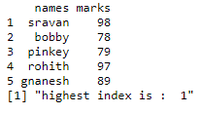If there is more than one maximum value, then it will return index of first number which is repeated.

Example 2:

## R

 `# vector 1``data1=``c``(``"sravan"``,``"bobby"``,``"pinkey"``,``"rohith"``,``        ``"gnanesh"``,``'divya'``,``"satwik"``,``"chandu"``)`` ` `# vector 2``data2=``c``(98,78,79,97,89,89,99,99)`` ` `# creating a dataframe with names and marks ``# using above vectors``final <- ``data.frame``(names=data1,marks=data2)``                    ` `print``(final)`` ` `print``(``paste``(``"highest index is : "``,``which.max``(final\$marks)))`

Output: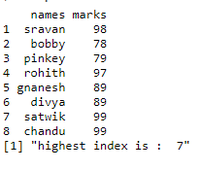If the data is of type character, it will find the maximum value using ASCII values.

Example 3:

## R

 `# vector 1``data1=``c``(``"sravan"``,``"bobby"``,``"pinkey"``,``"rohith"``,``        ``"gnanesh"``,``'divya'``,``"satwik"``,``"zhandu"``)`` ` `# vector 2``data2=``c``(98,78,79,97,89,89,99,99)`` ` `# creating a dataframe with names and marks ``# using above vectors``final <- ``data.frame``(names=data1,marks=data2)``                    ` `print``(final)`` ` `# display maximum value index for character values``print``(``paste``(``"highest index is : "``,``which.max``(final\$names)))`

Output: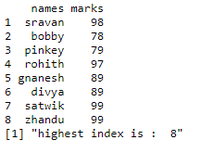Example 4:

## R

 `# vector 1 that contains NA values as characters``data1=``c``(``NA``,``"sravan"``,``NA``,``NA``,``NA``)`` ` `# vector 2 comtains all data``data2=``c``(102,98,98,102,102)`` ` `# creating a dataframe with names and marks``# using above vectors``final <- ``data.frame``(names=data1,marks=data2)``                    ` `print``(final)`` ` `# display maximum value index for character values``print``(``paste``(``"highest index is : "``,``which.max``(final\$names)))`` ` `# display maximum value index for  marks values``print``(``paste``(``"highest index is : "``,``which.max``(final\$marks)))`

Output: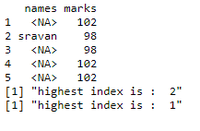If row containing all the values are same so all are higher. So it will return index of first element.

Example 5:

## R

 `# vector contains all same data``data2=``c``(102,102,102,102,102)`` ` `# creating a dataframe marks using above vector``final <- ``data.frame``(marks=data2)``                    ` `print``(final)`` ` `# display maximum value index for  marks values``print``(``paste``(``"highest index is : "``,``which.max``(final\$marks)))`

Output: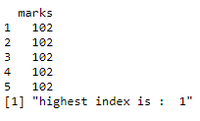If the data contains values as NA, then it will return empty.

Example 6:

## R

 `# vector contains all NA's``data2=``c``(``NA``,``NA``)`` ` `# creating a dataframe marks using``# above vector``final <- ``data.frame``(marks=data2)``                    ` `print``(final)`` ` `# display maximum value index for  marks values``print``(``paste``(``"highest index is : "``,``which.max``(final\$marks)))`

Output: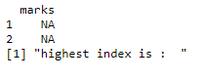My Personal Notes arrow_drop_up